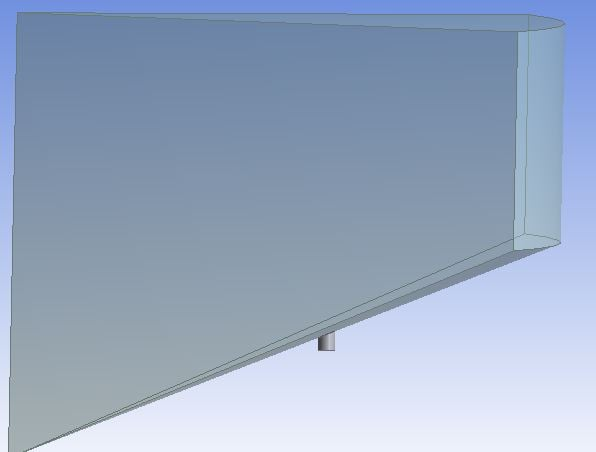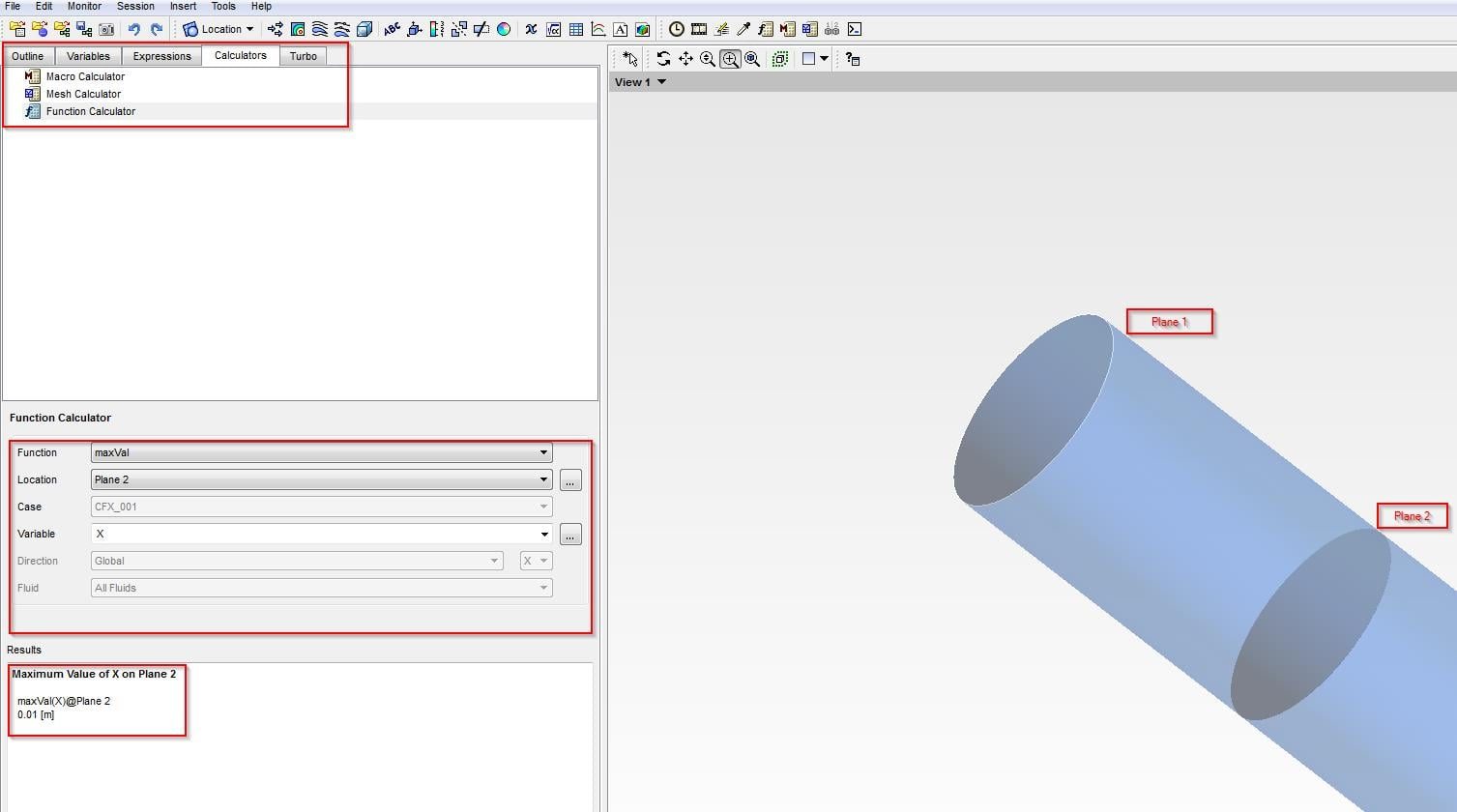## Fluids

•bushrar
Subscriber

Hello,

I was wondering if there was a way to obtain the coordinates of any part on the geometry without having to go through a process of trial and error?

Thanks!

bushrar

•raul.raghav
Subscriber

Can you explain what you are exactly trying to do? A picture would always help.

But from what I understand, I think you are trying to probe a certain location (region of max variable value or min variable value) and get the X, Y & Z locations. And what you are asking is how to find the coordinate points of that certain location? If that's what you are intending to do, you can:

1. Create a "Point 1" with "Variable Minimum" or "Variable Maximum" and select the variable of interest. Now go to the "Calculators" tab --> "Function Calculator", "Probe" --> "Point 1" and select "X", "Y" and "Z" as the variable to get the coordinate points.

If you have many such points, you can: "File" --> "Export" --> "Locations: Point 1" and "Save". Make sure the "Export Geometry Information" box checked. The exported .csv file have the X, Y and Z data along with the variable data at that point.

Hope this helps. Good luck!

•bushrar
Subscriber

raul.raghav,

Specifically what I was trying to do then was find  coordinates at the start and end of the capillary, for confirming its length, on the middle of the cone as shown below:When I create 'Point 1' do I just leave it with those random default coordinates or do I need to adjust such that it is at least close to the point of interest?

Thanks!

Bushra

•raul.raghav
Subscriber

For geometry based features like coordinate points and length, it would be a lot easier if you used Spaceclaim (or whichever software you're using to make the geometry). However, if you want to measure the length in CFD-Post, it would be easier if you can create planes at the two locations (start and end of the capillary, in your case) and using

Calculators --> Function Calculator --> maxVal --> Plane 1 --> X/Y/Z. (Select the appropriate coordinate axis that is aligned along the length).

If you have two planes, you can evaluate the "X/Y/Z" for the two planes and the difference would be the length that you would be interested in. Hope this helps! I'd still recommend you to do the length calculation using the software that you use to create the geometry. Attached is a screenshot of how it can be done: